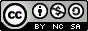Journal topic
Nonlin. Processes Geophys., 3, 143–149, 1996
https://doi.org/10.5194/npg-3-143-1996Nonlin. Processes Geophys., 3, 143–149, 1996
https://doi.org/10.5194/npg-3-143-199630 Sep 1996

30 Sep 1996

# The quasi-static approximation of the spring-slider motion

M. E. Belardinelli1,* and E. Belardinelli2 M. E. Belardinelli and E. Belardinelli
• 1Dipartimento di Fisica, Università di Bologna, Italy
• 2Dipartimento di Elettronica, Informatica e Sistemistica, Università di Bologna, Italy
• *Present address Istituto Nazionale di Geofisica, Roma, Italy

Abstract. The spring-slider is a simple dynamical system consisting in a massive block sliding with friction and pulled through a spring at a given velocity. Understanding the block motion is fundamental for studying more complex phenomena of frictional sliding, such as the seismogenic fault motion. We analyze the dynamical properties of the system, subject to rate- and state-dependent friction laws and forced at a constant load velocity. In particular we study the limits within which the quasi-static model can be used. The latter model approximates the complete model of the system without taking into account the inertia effects. The system parameters are here found to be grouped into three characteristic times of the three dynamics present in the complete model. A necessary condition for the quasi-static approximation to hold is that the characteristic time of the inertial equation is much smaller than the other two characteristic times. We have studied a modification of one of the classical forms of the rate- and state-dependent friction laws. Subsequently we have developed a linear analysis in the neighbourhood of the equilibrium point of the system. For the quasi-static model we rigorously found, by means of a nonlinear analysis, a supercritical Hopf bifurcation, a dynamical property of the complete model. The classical form of the friction laws can be obtained as a particular case of the one we considered, but fails to preserve the Hopf bifurcation in the quasi-static approximation. We conclude that to have a good quasi-static approximation of the system, even in nonlinear conditions, the form of the friction laws considered is a critical factor.